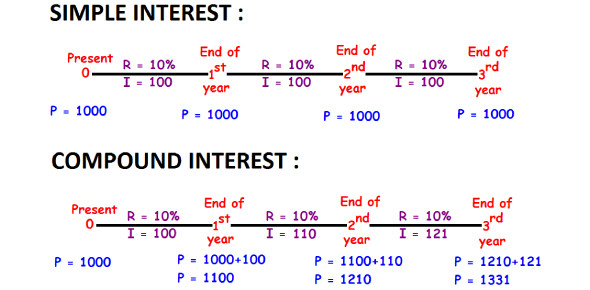# Quiz: Simple And Compound Interest

12 Questions | Attempts: 2733
ShareSettingsAre you preparing for any competitive exam and simple and compound interest going over your head? If yes, take this informative quiz to check your preparation level. Compound interest, at time, can be very confusing and complex. This quiz will provide you practice set that will help you gain mastery over the complex concepts. Take this quiz and gauge your understanding of the topic. If you find the quiz helpful, do share it with your friends. All the best!

• 1.
A father left a will of Rs.35 lakhs between his two daughters aged 8.5 and 16 such that they may get equal amounts when each of them reach the age of 21 years. The original amount of Rs.35 lakhs has been instructed to be invested at 10% p.a. simple interest. How much did the elder daughter get at the time of the will?
• A.

Rs. 17.5 lakhs

• B.

Rs. 21 lakhs

• C.

Rs. 15 lakhs

• D.

Rs. 20 lakhs

• 2.
What will Rs.1500 amount to in three years if it is invested in 20% p.a. compound interest, interest being compounded annually?
• A.

2400

• B.

2678

• C.

2592

• D.

2540

• 3.
If a sum of money grows to 144/121 times when invested for two years in a scheme where interest is compounded annually, how long will the same sum of money take to treble if invested at the same rate of interest in a scheme where interest is computed using simple interest method?
• A.

9 years

• B.

22 years

• C.

18 years

• D.

33 years

• 4.
The population of a town was 3600 three years back. It is 4800 right now. What will be the population three years down the line, if the rate of growth of population has been constant over the years and has been compounding annually?
• A.

6000

• B.

6400

• C.

7200

• D.

9600

• 5.
A man invests Rs.5000 for 3 years at 5% p.a. compound interest reckoned yearly. Income tax at the rate of 20% on the interest earned is deducted at the end of each year. Find the amount at the end of the third year
• A.

5624.32

• B.

5630.50

• C.

5788.125

• D.

5627.20

• 6.
The difference between the compound interest and the simple interest on a certain sum at 12% p.a. for two years is Rs.90. What will be the value of the amount at the end of 3 years?
• A.

9000

• B.

6250

• C.

8530.80

• D.

8780.80

• 7.
Vijay invested Rs.50,000 partly at 10% and partly at 15%. His total income after a year was Rs.7000. How much did he invest at the rate of 10%?
• A.

Rs.45,000

• B.

Rs.40,000

• C.

Rs.12,000

• D.

Rs.20,000

• 8.
A sum of money invested for a certain number of years at 8% p.a. simple interest grows to Rs.180. The same sum of money invested for the same number of years at 4% p.a. simple interest grows to Rs.120 only. For how many years was the sum invested?
• A.

25 years

• B.

40 years

• C.

33 years and 4 months

• D.

Cannot be determined

• 9.
How long will it take for a sum of money to grow from Rs.1250 to Rs.10,000, if it is invested at 12.5% p.a simple interest?
• A.

8 years

• B.

64 years

• C.

72 years

• D.

56 years

• 10.
Rs. 5887 is divided between Shyam and Ram, such that Shyam's share at the end of 9 years is equal to Ram's share at the end of 11 years, compounded annually at the rate of 5%. Find the share of Shyam
• A.

2088

• B.

2000

• C.

3087

• D.

None of these

• 11.
The question for the day is from the topic simple and compound interest. Shawn invested one half of his savings in a bond that paid simple interest for 2 years and received Rs.550 as interest. He invested the remaining in a bond that paid compound interest, interest being compounded annually, for the same 2 years at the same rate of interest and received Rs.605 as interest. What was the value of his total savings before investing in these two bonds?
• A.

Rs.5500

• B.

Rs.11000

• C.

Rs.22000

• D.

Rs.2750

• 12.
Rs.100 doubled in 5 years when compounded annually. How many more years will it take to get another Rs.200 compound interest?
• A.

10 years

• B.

5 years

• C.

7.5 years

• D.

15 years

## Related TopicsBack to top
×

Wait!
Here's an interesting quiz for you.